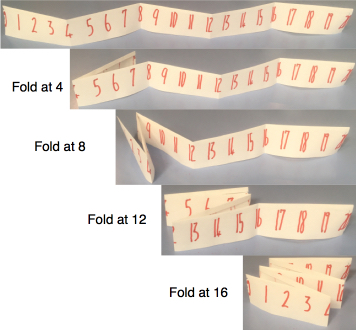#### You may also like### Prompt Cards

These two group activities use mathematical reasoning - one is numerical, one geometric.### Consecutive Numbers

An investigation involving adding and subtracting sets of consecutive numbers. Lots to find out, lots to explore.### Exploring Wild & Wonderful Number Patterns

EWWNP means Exploring Wild and Wonderful Number Patterns Created by Yourself! Investigate what happens if we create number patterns using some simple rules.

# Folded Number Line

##### Age 7 to 11Challenge Level

I made a number line from 0 to 20 and folded it as shown below:I folded it along the 4 and the 8 so that the 0 is on top of the 8 and the 4 is on top of the 12.

I then folded it along the 12 and the 16 so that now the 0 is on top of 8 which is on top of 16 and the 4 is on top of the 12 which is on top of the 20.

I ended up with some stacks of numbers that are on top of each other.

For example:
• 1, 7, 9, 15 and 17 make a stack which has total of 49.
• The stack made of 0, 8 and 16 has a total of 24.
• The stack made of 3, 5, 11, 13 and 19 has a total of 51.
We will be focusing on totals made in this way (like 49, 24 and 51 above).

### The challenges below involve varying the length of the number line. However, you must always start with 0 and each folded section must be the same length. There must always be at least one fold in the number line and folds must go through a number.

#### Challenge 1

Find three different ways to get a 'stack total' of 36 using lengths of 21 to 36 inclusive, showing exactly how you fold the number lines.

#### Challenge 2

Find as many ways as you can to get a 'stack total' of 48 using lengths of 21 to 36 inclusive, again showing exactly how you fold the number lines.

#### Challenge 3

Now using lengths from 21 to 50 inclusive, find a length which contains all the 'stack totals' of 69, 70 and 71 when folded.

This sheet contains a copy of the task.